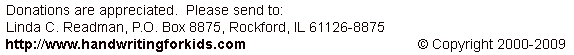Web www.handwritingforkids.com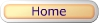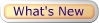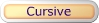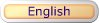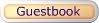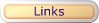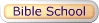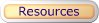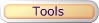• Online / Interactive
• Printable - w/guideline
• Printable
• Customized

This online/interactive visual math addition, will create 1 digit by 1 digit (1x1) picture only equations, and it will automatically graded/scored your results in a printable worksheet. No Timed-Test.

 Type of online math problems: Online - Visual Math - No Carryovers Online - Visual Math - Carryovers Online - Visual Math - Addition: 0 to 5 Online - Visual Math - Addition: 5 to 10 Online - Visual Math - Addition: 0 to 10 Online - Visual Math - Addition: 0 to 18 Online - Visual Math - Addition: 10 to 18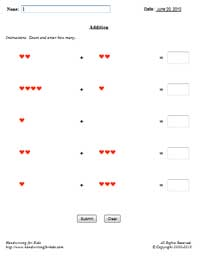This math worksheet maker will create a horizontal visual math addition, 1 digit by 1 digit (1x1) picture only equations with the handwriting lines.

 See sample math worksheets below:

This math worksheet maker will create a horizontal visual math addition, 1 digit by 1 digit (1x1) picture only equations without the handwriting lines.

 See sample math worksheets below:
 This worksheet generator will create your own customized/personalized visual math addition worksheet. You enter your own math problems to create your own picture only printable worksheet. Addend ranges between 0 and 20 only.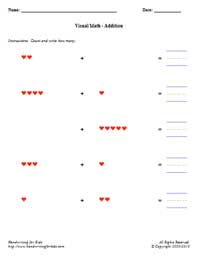• Online / Interactive
• Printable - w/guideline
• Printable
• Customized

This online/interactive math addition generator will provides plenty of online math practice, boost test scores and prepare the child/children to meet the Kindergarten, Grade 1, and Grade 2 curriculum standards. This online/interactive math addition, will create the vertical 1 digit by 1 digit (1x1) equations with an option of timed test, and it will automatically graded/scored your results in a printable worksheet.

Type of online math problems:

• Online - Addition - No Carryovers
• Online - Addition - Carryovers
 1 +  3 4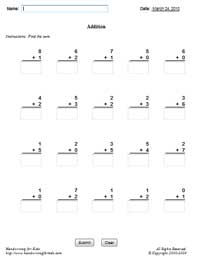This printable basic math addition worksheet generator will provides plenty of math practice, boost test scores and prepare the child/children to meet the Kindergarten, Grade 1, and Grade 2 curriculum standards. This math worksheet maker will create the vertical math addition, 1 digit by 1 digit (1x1) equations with handwriting lines.

See sample math worksheets below:

 3 +  6 9

This printable basic math addition worksheet generator will provides plenty of math practice, boost test scores and prepare the child/children to meet the Kindergarten, Grade 1, and Grade 2 curriculum standards. This math worksheet maker will create the vertical math addition, 1 digit by 1 digit (1x1) equations.

See sample math worksheets below:

 4 +  1 5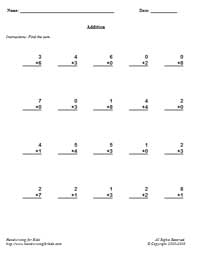This worksheet generator will create your own customized/personalized addition worksheet. You enter your own vertical math problems in a printable worksheet. Maximum 5 digits x 5 digits (5x5).

 4 +  2 8• Online / Interactive
• Printable - w/guideline
• Printable
• Customized

This online/interactive math addition generator will provides plenty of online math practice, boost test scores and prepare the child/children to meet the Grade 1, Grade 2, and Grade 3 curriculum standards. This online/interactive math addition, will create the vertical 2 digits by 1 digit (2x1) and 1 digit by 2 digits (1x2) equations with an option of timed test, and it will automatically graded/scored your results in a printable worksheet.

Type of online math problems:

• Addition - No Carryovers (2 Digits - Top Only)
• Addition - No Carryovers (2 Digits - Bottom Only)
• Addition - No Carryovers (2 Digits - Random)
• Addition - Carryovers (2 Digits - Top Only)
• Addition - Carryovers (2 Digits - Bottom Only)
• Addition - Carryovers (2 Digits - Random)
 63 +  8 71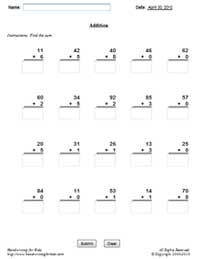This printable basic math addition worksheet generator will provides additional of math practice, boost test scores and prepare the child/children to meet the Grade 1, Grade 2, and Grade 3 curriculum standards. This math worksheet maker will create the vertical math addition, 2 digits by 1 digit (2x1), and 1 digit by 2 digits (1x2) equations with handwriting lines.

See sample math worksheets below:

 21 +  4 25

This printable basic math addition worksheet generator will provides additional of math practice, boost test scores and prepare the child/children to meet the Grade 1, Grade 2, and Grade 3 curriculum standards. This math worksheet maker will create the vertical math addition, 2 digits by 1 digit (2x1), and 1 digit by 2 digits (1x2) equations without the handwriting lines.

See sample math worksheets below:

 16 +  5 21

This worksheet generator will create your own customized/personalized addition worksheet. You enter your own vertical math problems in a printable worksheet. Maximum 5 digits x 5 digits (5x5).

 58 +  3 61• Online / Interactive
• Printable w/guideline
• Printable
• Customized

This online/interactive math addition generator will provides plenty of online math practice, boost test scores and prepare the child/children to meet the Kindergarten, Grade 1, and Grade 2 curriculum standards. This online/interactive math addition, will create the horizontal 1 digit by 1 digit (1x1) equations with an option of timed test, and it will automatically graded/scored your results in a printable worksheet.

 4 + 1 = 5This printable basic math addition worksheet generator will provides plenty of math practice, boost test scores and prepare the child/children to meet the Kindergarten, Grade 1, and Grade 2 curriculum standards. This math worksheet maker will create the horizontal math addition, 1 digit by 1 digit (1x1) equations with handwriting lines.

 8 + 6 = 14

 See sample math worksheets below:

This printable basic math addition worksheet generator will provides plenty of math practice, boost test scores and prepare the child/children to meet the Kindergarten, Grade 1, and Grade 2 curriculum standards. This math worksheet maker will create the horizontal math addition, 1 digit by 1 digit (1x1) equations without handwriting lines.

 3 + 5 = 8

 See sample math worksheets below:

This worksheet generator will create your own customized/personalized addition worksheet. You enter your own vertical math problems in a printable worksheet. Maximum 5 digits x 5 digits (5x5).

 73 + 6 = 79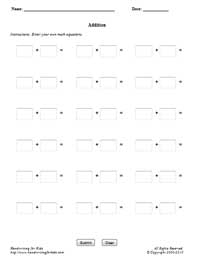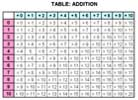Examples Addition Tables How to use the addition table Blank Table #1 Answer SheetAddition 0-12 Flash Cards (MS Word Document - Printable Only) Flash Cards Printing Instructions: 1. Print all odd pages 2. Flip the stack of printed pages and re-insert into the printer. 3. Print all even pages. Suggestion: Print on color paper or card-stock paper.

### JavaScript Interactive Math* - Simple Addition

 Level 1: Visual Math Hearts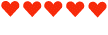Dominoes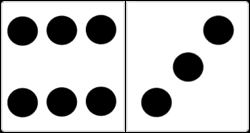Level 2: Addition - 1 Digit by 1 Digit (No Carryovers)
 Exercise 1 Exercise 6 Exercise 11 Exercise 16 Exercise 2 Exercise 7 Exercise 12 Exercise 17 Exercise 3 Exercise 8 Exercise 13 Exercise 18 Exercise 4 Exercise 9 Exercise 14 Exercise 19 Exercise 5 Exercise 10 Exercise 15 Exercise 20
 1 +  2 3

1 Digit by 1 Digit
Level 2: Addition - 2 Digits by 1 Digit (No Carryovers)
 Exercise 1 Exercise 6 Exercise 11 Exercise 16 Exercise 2 Exercise 7 Exercise 12 Exercise 17 Exercise 3 Exercise 8 Exercise 13 Exercise 18 Exercise 4 Exercise 9 Exercise 14 Exercise 19 Exercise 5 Exercise 10 Exercise 15 Exercise 20
 10 + 2 12

2 Digits by 1 Digit

Level 3: Addition - 1 Digit by 1 Digit (Carryovers)
 Exercise 1 Exercise 6 Exercise 11 Exercise 16 Exercise 2 Exercise 7 Exercise 12 Exercise 17 Exercise 3 Exercise 8 Exercise 13 Exercise 18 Exercise 4 Exercise 9 Exercise 14 Exercise 19 Exercise 5 Exercise 10 Exercise 15 Exercise 20
 9 +  2 11

1 Digit by 1 Digit
Level 3: Addition - 2 Digits by 1 Digit (Carryovers)
 Exercise 1 Exercise 6 Exercise 11 Exercise 16 Exercise 2 Exercise 7 Exercise 12 Exercise 17 Exercise 3 Exercise 8 Exercise 13 Exercise 18 Exercise 4 Exercise 9 Exercise 14 Exercise 19 Exercise 5 Exercise 10 Exercise 15 Exercise 20
 18 + 5 23

2 Digits by 1 Digit

30 Problems - Worksheets
Some may contained carryovers and non-carryovers
1 Digit by 1 Digit
2 Digits by 1 Digit
2 Digits by 2 Digits
 1 +  2 3

 10 + 2 12
 13 +25 38

* JavaScript must be enabled on your browser.

Sudoku for Kids (Picture Sudoku) 4x4